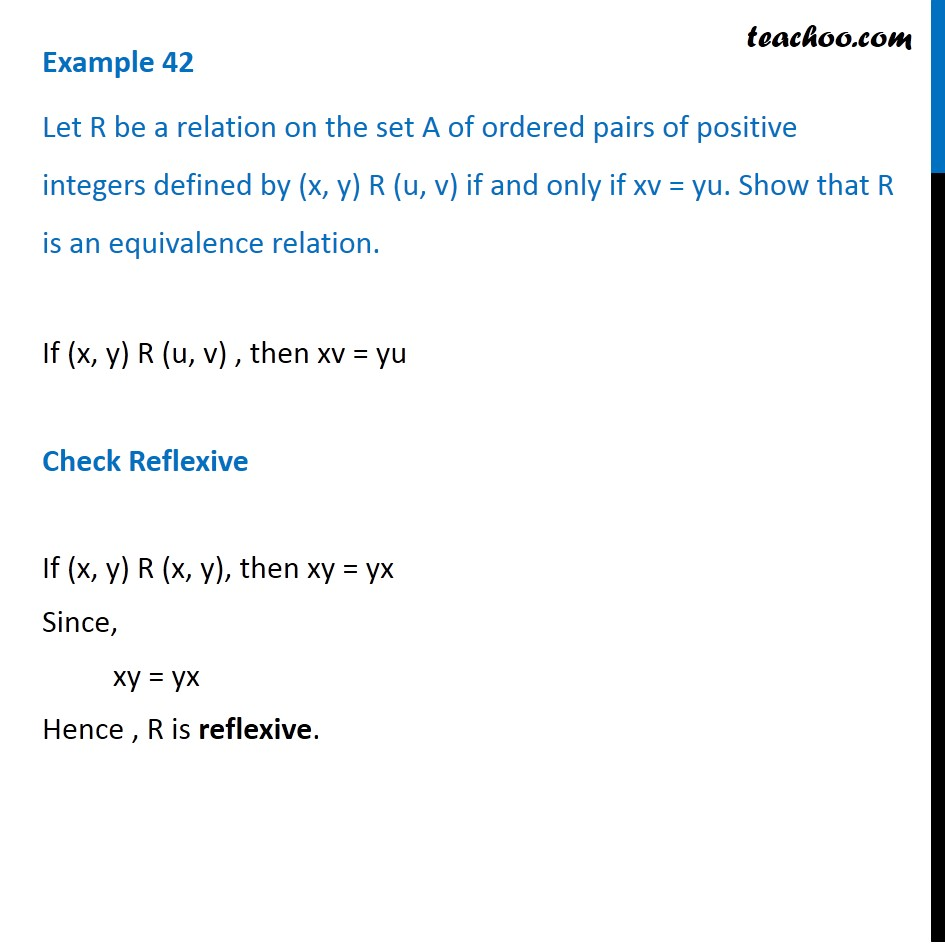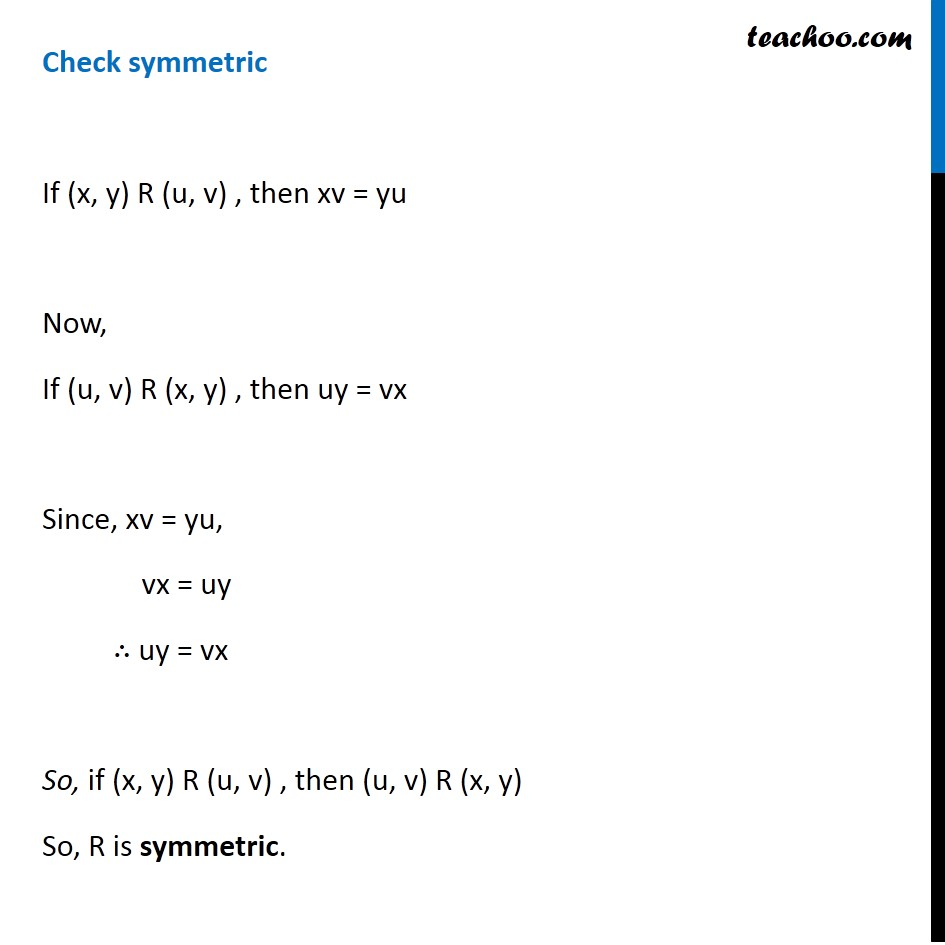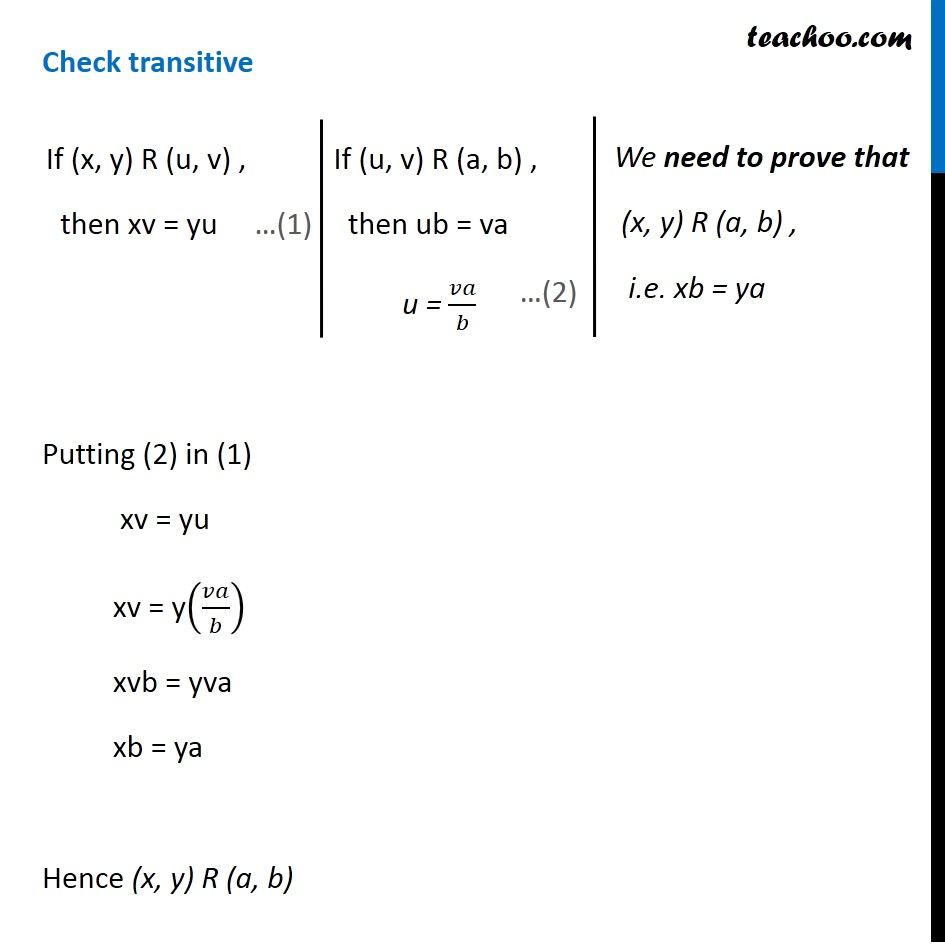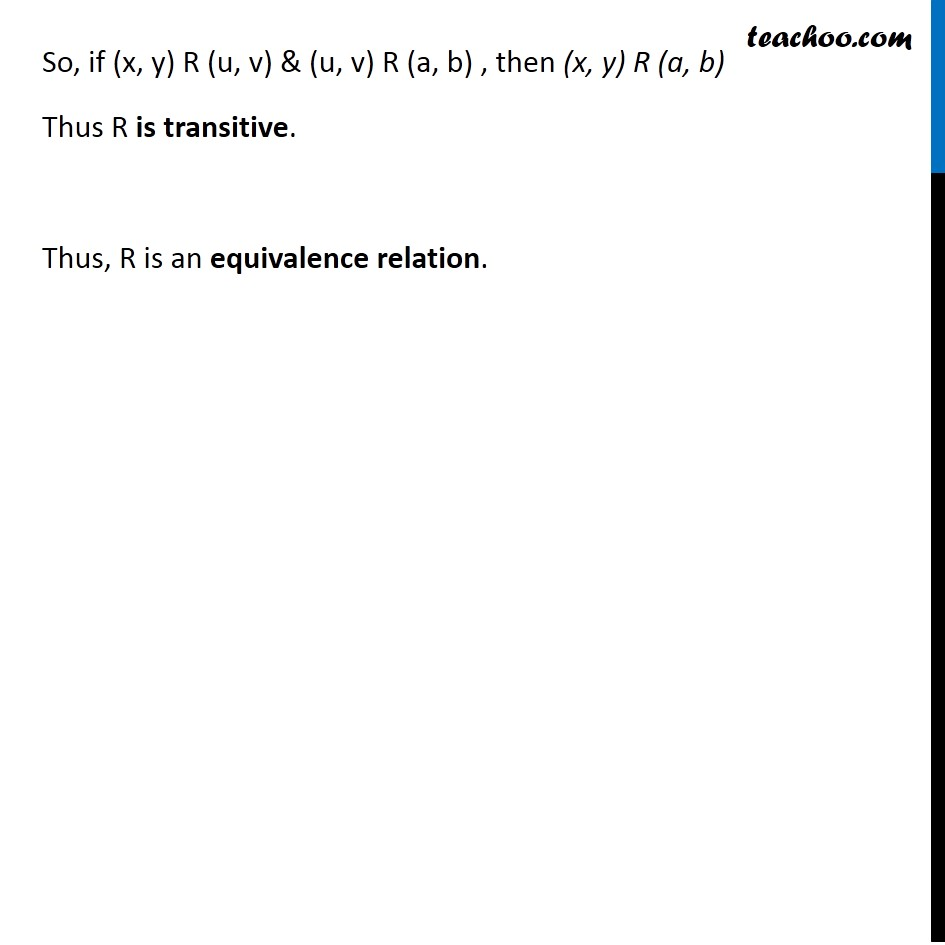To prove relation reflexive, transitive, symmetric and equivalent

Chapter 1 Class 12 Relation and Functions
Concept wiseIntroducing your new favourite teacher - Teachoo Black, at only ₹83 per month

### Transcript

Example 42 Let R be a relation on the set A of ordered pairs of positive integers defined by (x, y) R (u, v) if and only if xv = yu. Show that R is an equivalence relation. If (x, y) R (u, v) , then xv = yu Check Reflexive If (x, y) R (x, y), then xy = yx Since, xy = yx Hence , R is reflexive. Check symmetric If (x, y) R (u, v) , then xv = yu Now, If (u, v) R (x, y) , then uy = vx Since, xv = yu, vx = uy ∴ uy = vx So, if (x, y) R (u, v) , then (u, v) R (x, y) So, R is symmetric. If (x, y) R (u, v) , then xv = yu If (u, v) R (a, b) , then ub = va u = 𝑣𝑎/𝑏 We need to prove that (x, y) R (a, b) , i.e. xb = ya We need to prove that (x, y) R (a, b) , i.e. xb = ya Check transitive Putting (2) in (1) xv = yu xv = y(𝑣𝑎/𝑏) xvb = yva xb = ya Hence (x, y) R (a, b) So, if (x, y) R (u, v) & (u, v) R (a, b) , then (x, y) R (a, b) Thus R is transitive. Thus, R is an equivalence relation.